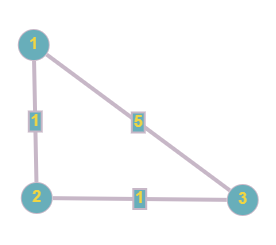Problem title
Difficulty
Avg time to solve

Repeat Triangle
Easy
15 mins
MegaPrime Numbers
Easy
15 mins
Mirror image of triangle
Easy
10 mins
Maximum Difference
Moderate
30 mins
Magician and Chocolates
Easy
15 mins
Possible Balanced Strings
Moderate
15 mins
Maximum value node
Easy
15 mins
Maximum 0-1 Distance
Hard
10 mins
Next Higher Node
Easy
15 mins
Path Queries
Moderate
25 mins2

# Path Queries

Difficulty: MEDIUM
Avg. time to solve
25 min
Success Rate
70%

Problem Statement

#### You have to answer ‘Q’ queries. Every query has two integers, ‘u’, ‘v’, representing the vertices. Your task is to print the shortest distance between ‘u’ and ‘v’ and print -1 if there is no path.

##### For example:``````For the given graph, we have to find the shortest distance between vertices 1 and 3.
The shortest distance between 1 and 3 is 2 (via 1 <-> 2<-> 3). Hence, the answer is 2.
``````
##### Input Format:
``````The first line of the input contains an integer, 'T,’ denoting the number of test cases.

The first line of each test case contains three integers ‘V’, ‘E’, ‘Q’, denoting the number of vertices, the number of edges in the graph, and the number of queries.

The Next ‘E’ lines of each test case have three integers ‘u’,’v’,’w’ corresponding to vertices ‘u’ and ‘v’ are connected with a weight ‘w’.

The Next ‘Q’ lines of each test case have two integers, ‘u’,’v’ representing two vertices.
``````
##### Output Format:
``````For each test case, print ‘Q’ lines, each line has one integer corresponding to the minimum distance for the given query.

Print the output of each test case in a separate line.
``````
##### Note:
``````You do not need to print anything. It has already been taken care of. Just implement the given function.
``````
##### Constraints:
``````1 <= T <= 5
1 <= V <= 100
1 <= E <= (V *(V - 1)) / 2
1 <= Q <= 10^5
1 <= u , v <= V
1 <= w <= 10^6

Time limit: 1 sec
``````
##### Sample Input 1:
``````2
3 3 1
1 2 1
2 3 1
1 3 5
1 3
4 3 2
1 2 1
2 3 5
1 3 3
2 4
1 3
``````
##### Sample Output 1:
``````2
-1
3
``````
##### Explanation of sample input 1:
``````For the first test case,
The shortest distance between 1 and 3 is 2 (via 1 <-> 2<-> 3). Hence, the answer is 2.

For the second test case,
For the first query, -1 because vertex 4 is unreachable from vertex 2. Hence, the answer is -1.
For the second query, The shortest distance between 1 and 3 is 3 (via 1<-> 3). Hence, the answer is 3.
``````
##### Sample Input 2:
``````2
4 3 1
1 2 1
2 3 10
4 1 5
1 1
4 2 2
1 3 3
1 2 1
2 3
4 2
``````
##### Sample Output 2:
``````0
4
-1
``````Console